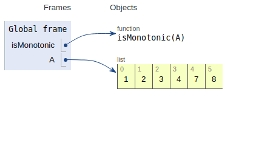# Python Program to check if the given array is Monotonic

In this article, we will learn about the solution and approach to solve the given problem statement.

## Problem statement

Given an array input Arr containing n integers. We need to check whether the input array is Monotonic in nature or not.

An array is said to be monotonic in nature if it is either continuously increasing or continuously decreasing.

Mathematically,

An array A is continuously increasing if for all i <= j,

A[i] <= A[j].

An array A is continuously decreasing if for all i <= j,

A[i] >= A[j].

Here we will check whether all the adjacent elements satisfy one of the above conditions or not.

Now let’s see the implementstion −

## Example

Live Demo

def isMonotonic(A):
return (all(A[i] <= A[i + 1] for i in range(len(A) - 1)) or
all(A[i] >= A[i + 1] for i in range(len(A) - 1)))
# main
A = [1,2,3,4,7,8]
print(isMonotonic(A))

## Output

True

All the variables are declared in the global frame as shown in the figure given below −## Conclusion

In this article, we learnt about the approach to find whether an array is monotonic in nature or not